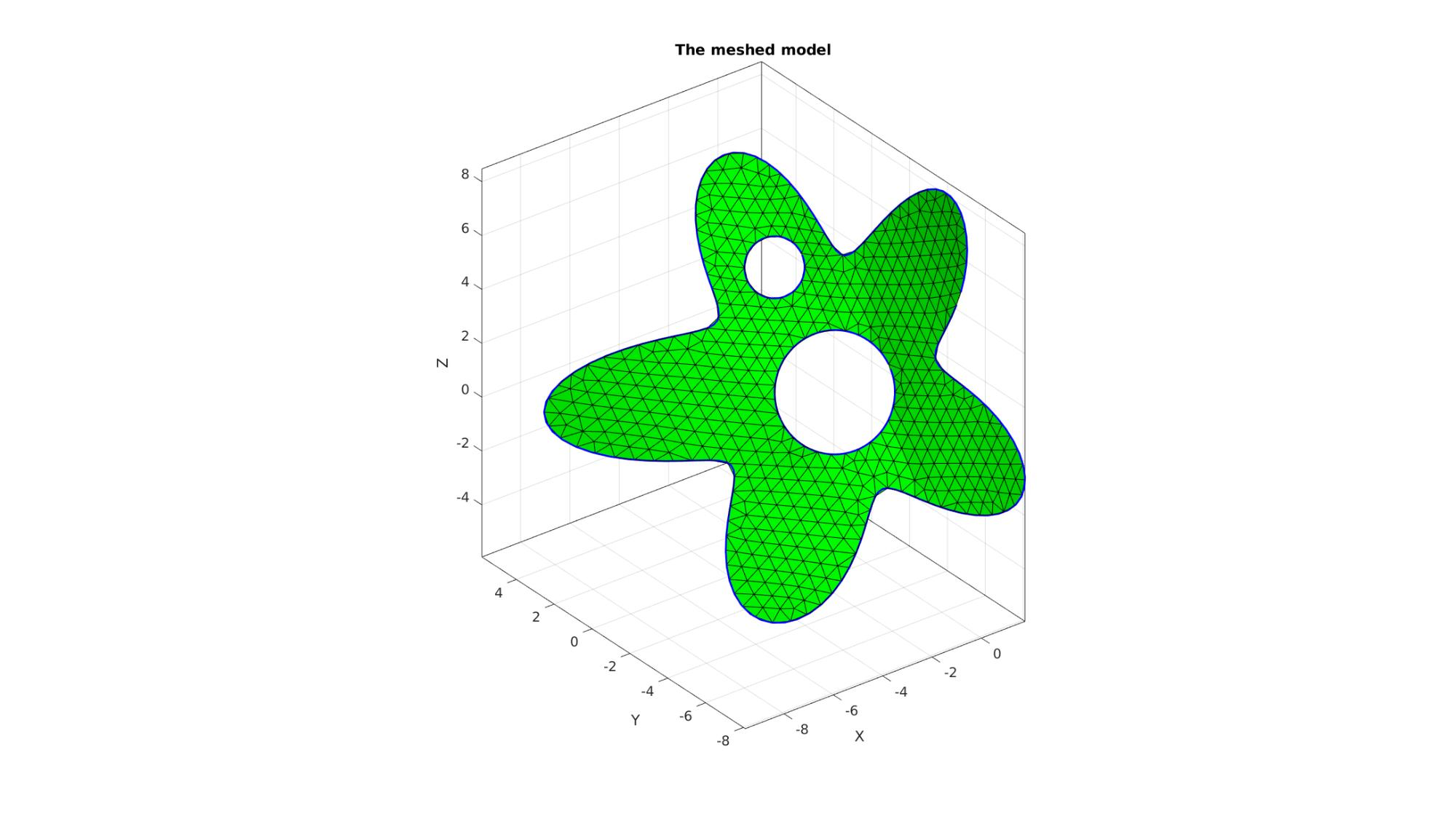# regionTriMesh3D

Below is a basic demonstration of the features of the regionTriMesh3D function.

## Syntax

[F,V]=regionTriMesh3D(regionCell,pointSpacing,resampleCurveOpt,interpMethod);

## Description

This function meshes a 3D region. It is conceptually similar to regionTrimMesh2D. The 3D regions are rigidly transformed to a nearly 2D form and regionTrimMesh2D is then used for 2D meshing. Then interpolation is to get the coordinates in the 3rd dimension.

## Examples

```clear; close all; clc;
```

Plot settings

```fontSize=15;
markerSize1=45;
lineWidth1=4;
faceAlpha=0.5;
```

## Example: Meshing a 3D region

Creating example boundary curves and input region specification

```%Boundary 1
ns=150;
t=linspace(0,2*pi,ns);
t=t(1:end-1);
r=6+2.*sin(5*t);
[x,y] = pol2cart(t,r);
z=1/10*x.^2;
V1=[x(:) y(:) z(:)];

%Boundary 2
ns=100;
t=linspace(0,2*pi,ns);
t=t(1:end-1);
[x,y] = pol2cart(t,ones(size(t)));
z=zeros(size(x));
V2=[x(:) y(:)+4 z(:)];

%Boundary 3
ns=75;
t=linspace(0,2*pi,ns);
t=t(1:end-1);
[x,y] = pol2cart(t,2*ones(size(t)));
z=zeros(size(x));
V3=[x(:) y(:)-0.5 z(:)];

%Create Euler angles to set directions
E=[0.25*pi -0.25*pi 0];
[R,~]=euler2DCM(E); %The true directions for X, Y and Z axis

V1=(R*V1')'; %Rotate polygon
V2=(R*V2')'; %Rotate polygon
V3=(R*V3')'; %Rotate polygon

regionCell={V1,V2,V3}; %A region between V1 and V2 (V2 forms a hole inside V1)
```

```%Defining a region and control parameters (See also |regionTriMesh2D|)
pointSpacing=0.5; %Desired point spacing
resampleCurveOpt=1;
interpMethod='linear'; %or 'natural'
[F,V]=regionTriMesh3D(regionCell,pointSpacing,resampleCurveOpt,interpMethod);
```

Plotting meshed model

```hf1=cFigure;
title('The meshed model','FontSize',fontSize);
xlabel('X','FontSize',fontSize);ylabel('Y','FontSize',fontSize); zlabel('Z','FontSize',fontSize);
hold on;

patch('Faces',F,'Vertices',V,'FaceColor','g');

plotV(V1,'b-','LineWidth',2);
plotV(V2,'b-','LineWidth',2);
plotV(V3,'b-','LineWidth',2);

axis equal; view(3); axis tight;  grid on;  set(gca,'FontSize',fontSize);
drawnow;
```GIBBON www.gibboncode.org

Kevin Mattheus Moerman, [email protected]

GIBBON footer text

GIBBON: The Geometry and Image-based Bioengineering add-On. A toolbox for image segmentation, image-based modeling, meshing, and finite element analysis.

Copyright (C) 2019 Kevin Mattheus Moerman

This program is free software: you can redistribute it and/or modify it under the terms of the GNU General Public License as published by the Free Software Foundation, either version 3 of the License, or (at your option) any later version.

This program is distributed in the hope that it will be useful, but WITHOUT ANY WARRANTY; without even the implied warranty of MERCHANTABILITY or FITNESS FOR A PARTICULAR PURPOSE. See the GNU General Public License for more details.

You should have received a copy of the GNU General Public License along with this program. If not, see http://www.gnu.org/licenses/.